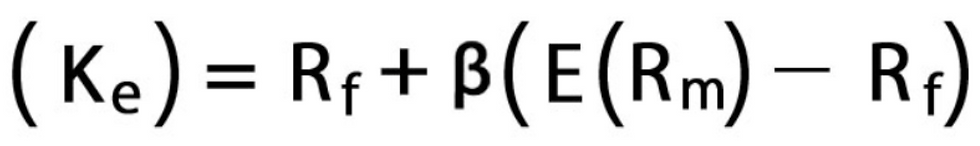top of page

# WACC / Cost of Equity / Unlevered VS Levered Free Cash Flow

By Victor Paquet (Mergersight Operations) and Carlo Lepoardi & Tommaso Arona (Boston University partners)

## Walkthrough

### WACC

The WACC, or Weighted Average Cost of Capital, is the discount factor you use in your DCF valuation to account for the Time Value of Money and discount the Free Cash Flows back to their present value. The formula is:E/D+E and D/D+E are respectively the portions of equity and debt, Re and Rd are the costs of equity and debt and (1-t) is the tax shield provided by the debt (t: corporate tax rate).

To calculate the cost of equity (Re), see after.

For other components of the formula, you usually look at comparable companies and the interest rates and yields issued by similar companies to get estimates.

### Cost of Equity

The Cost of Equity is a measure of how much return an equity investor would expect in return of his/her investment in the company.

Hence, you should expect a company with a smaller market capitalization to have a bigger Cost of Equity. Indeed, a \$100mn market cap company is expected to outperform the market and therefore be riskier than a \$1bn market cap company.

To calculate the Cost of Equity, you can use the CAPM (Capital Asset Pricing Model):### CAPM

Rf: The risk-free rate represents how much a 10-year or 20-year Government Treasury Bill should yield.

Beta is a measure of systematic risk. It gauges the tendency of the return of a security to move in parallel with the return of the stock market as a whole. In other words, it is a measure of sensitivity of security's volatility relative to the market's volatility. A beta of 1 indicates that the security's price tends to move with the market, a beta of 0means that regardless of any market movement, the value of the security remains unchanged. A beta of -1.0 means that the stock is inversely correlated to the market.

Finally, the Equity Risk Premium is the percentage by which stocks are expected to out-perform “risk-less” assets hence why you compute the difference between the Expected Return of the Market (E(Rm)) and the Risk-Free Rate(Rf).

### Unlevered VS Levered FCF

Levered FCF is the amount of cash left after paying for financial costs such as interest costs and operating expenses whereas Unlevered FCF doesn’t take the cost of capital into account. In other words, the key difference between both is expenses.

Another way to see it: considering leverage is another name for debt, Levered FCF is the cash flow that only belongs to equity holders while Unlevered FCF belongs to both the equity and the debt holders.

Considering this explanation and the definition of WACC which takes the entire capital structure into account, you will have to use Unlevered FCFs for your DCF analysis. Reversely, if you use the Levered FCF, then you will have to use your Cost of Equity as the discount factor.

bottom of page